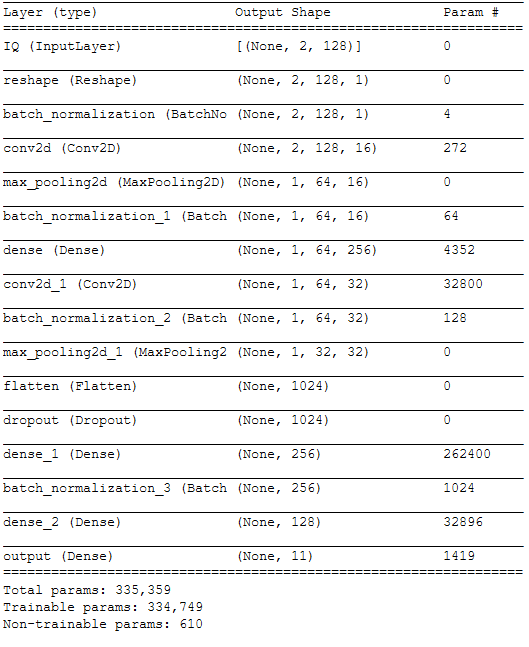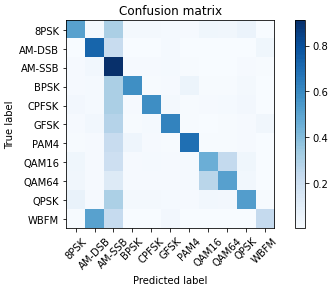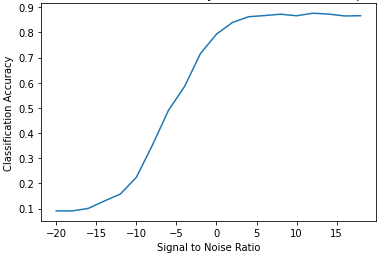## #StackBounty: #deep-learning #classification #keras #convolutional-neural-network #ai Convolutional Neural Network for Signal Modulatio…

### Bounty: 100

I recently posted another question and this question is the evolution of that one.

By the way I will resume all the problem below, like if the previous question didn’t ever exist.

# Problem description

I’m doing Signal Modulation Classification using a Convolutional Neural Network and I want to improve performance.

## Data

Dataset is composed by 220.000 rows like these. Data is perfectly balanced: I have 20.000 datapoints for each label.

Dataset column Type Range Form Notes
Signal i=real, q=real [i_0, i_1, …, i_n], [q_0, q_1, …, q_n] n=127
SNR s=integer [-18, 20] s
Label l=string l They are 11 labels

Lower is the SNR value, and noisier is the signal: classify low SNR signals is not that easy.

## Neural Network

Neural Network is a Convolutional Neural Network coded as below:

DROPOUT_RATE = 0.5

iq_in = keras.Input(shape=in_shp, name="IQ")
reshape = Reshape(in_shp + )(iq_in)
batch_normalization = BatchNormalization()(reshape)

conv_1 = Convolution2D(16, 4, padding="same", activation="relu")(batch_normalization)
batch_normalization_2 = BatchNormalization()(max_pool)
fc1 = Dense(256, activation="relu")(batch_normalization_2)
conv_2 = Convolution2D(32, 2, padding="same", activation="relu")(fc1)
batch_normalization_3 = BatchNormalization()(conv_2)

out_flatten = Flatten()(max_pool_2)
dr = Dropout(DROPOUT_RATE)(out_flatten)
fc2 = Dense(256, activation="relu")(dr)
batch_normalization_4 = BatchNormalization()(fc2)
fc3 = Dense(128, activation="relu")(batch_normalization_4)
output = Dense(11, name="output", activation="softmax")(fc3)

model = keras.Model(inputs=[iq_in], outputs=[output])

model.summary()## Training

Training is being done splitting the data in 75% as Training set, 25% as Test set.

NB_EPOCH = 100     # number of epochs to train on
BATCH_SIZE = 1024  # training batch size

filepath = NEURAL_NETWORK_FILENAME

history = model.fit(
X_train,
Y_train,
batch_size=BATCH_SIZE,
epochs=NB_EPOCH,
validation_data=(X_test, Y_test),
callbacks = [
keras.callbacks.ModelCheckpoint(filepath, monitor='val_loss', verbose=0, save_best_only=True, mode='auto'),
keras.callbacks.EarlyStopping(monitor='val_loss', patience=5, verbose=0, mode='auto')
])

# we re-load the best weights once training is finished


# ResultsMy evaluation system evaluate how accurate is my Neural Network for classifying signals with different SNR.# What did I try?

Thisis a list of things that I tried and I’m sure that are modifying performances in worse:

• Reducing batch size (only increases training time without improving test accuracy)
• Training without too noisy signals (lowers accuracy)
• Moving the Dropout layer before the Flatten layer

# Questions

Any suggestion to get better performances?

Get this bounty!!!

## #StackBounty: #unity #ai Agent trying to reach same position as other agents, instead start spinning in place

### Bounty: 50

I create units in my game and have a rally point where they should walk to when created, like Age of Empires.

My problem is that since they compete for this position after a while, sometimes they end up doing this:

https://gfycat.com/decentquestionableandeancondor

https://gfycat.com/densehonoredgalapagosalbatross

As you can see one of the guys cant reach the rally point, so he starts rotating.

I realize this has to do with stopping distance, but no matter how high I set the stopping distance this eventually becomes a problem when there are many enough units. And if I set it too high they dont walk to the rally point at all, since its not THAT far from the building they spawn from.

Is there any way to manage this scenario? i.e just making him go as close as he can, and then stop?

My AI Script:

using UnityEngine;
using UnityEngine.AI;

[RequireComponent(typeof(NavMeshAgent))]
public class BaseAi : MonoBehaviour
{
[HideInInspector]
public NavMeshAgent agent;

[HideInInspector]
public NavMeshObstacle obstacle;

float sampleDistance = 50;

Transform mTransform;

private void Awake()
{
agent = GetComponent<NavMeshAgent>();
obstacle = GetComponent<NavMeshObstacle>();
mTransform = transform;
}

private void Start()
{
agent.avoidancePriority = Random.Range(agent.avoidancePriority - 10, agent.avoidancePriority + 10);
}

void LateUpdate()
{
if (agent.isActiveAndEnabled && agent.hasPath)
{
var projected = agent.velocity;
projected.y = 0f;

if (!Mathf.Approximately(projected.sqrMagnitude, 0f))
mTransform.rotation = Quaternion.LookRotation(projected);
}
}

/// <summary>
/// Returns true if the position is a valid pathfinding position.
/// </summary>
/// <param name="position">The position to sample.</param>
public bool SamplePosition(Vector3 point)
{
NavMeshHit hit;
return NavMesh.SamplePosition(point, out hit, sampleDistance, NavMesh.AllAreas);
}

public bool SetDestination(Vector3 point)
{
if (SamplePosition(point))
{
agent.SetDestination(point);
return true;
}

return false;
}

public void Teleport(Vector3 point)
{
agent.Warp(point);
}

/// <summary>
/// Return true if agent reached its destination
/// </summary>
public bool ReachedDestination()
{
if (agent.isActiveAndEnabled && !agent.pathPending)
{
if (agent.remainingDistance <= agent.stoppingDistance)
{
if (!agent.hasPath || agent.velocity.sqrMagnitude == 0f)
{
return true;
}
}
}

return false;
}

public void ToggleObstacle(bool toggleOn)
{
agent.enabled = !toggleOn;
obstacle.enabled = toggleOn;
}

void OnDrawGizmos()
{
if (agent == null) { return; }
if (agent.path == null) { return; }

Color lGUIColor = Gizmos.color;

Gizmos.color = Color.red;
for (int i = 1; i < agent.path.corners.Length; i++)
{
Gizmos.DrawLine(agent.path.corners[i - 1], agent.path.corners[i]);
}
}
}


Get this bounty!!!

## #StackBounty: #unity #ai Unity – Moving large group of units takes too long, moving 1 by 1

### Bounty: 200

I use use Unity’s own NavMesh/AI system and I think it works pretty well. The only problem I have is that when I move large groups of units, they will find a path one by one, but it takes a really long time as you can see in the video:

https://gfycat.com/blankhalfdartfrog

To set destinations I use this:

    public bool SetDestination(Vector3 point)
{
if (SamplePosition(point))
{
agent.SetDestination(point);
return true;
}

return false;
}


I know I could wait until they all have pathPending == false, but that would mean the entire group moves with a huge delay. Is there some way I can improve or fix this issue?

Edit: It is quite simple to recreate (if you have some basic knowledge on Unity NavMesh), Simply create a “click to move”-script that moves a List of units to where you clicked using the code above (just fill the list in Start on each unit). Put down a few obstacles and ur done.

Get this bounty!!!

## Distributed Evolutionary Algorithms in Python

DEAP is a novel evolutionary computation framework for rapid prototyping and testing of ideas. It seeks to make algorithms explicit and data structures transparent. It works in perfect harmony with parallelization mechanism such as multiprocessing and SCOOP.

DEAP includes the following features:

• Genetic algorithm using any imaginable representation
• List, Array, Set, Dictionary, Tree, Numpy Array, etc.
• Genetic programing using prefix trees
• Loosely typed, Strongly typed
• Automatically defined functions
• Evolution strategies (including CMA-ES)
• Multi-objective optimisation (NSGA-II, SPEA2, MO-CMA-ES)
• Co-evolution (cooperative and competitive) of multiple populations
• Parallelization of the evaluations (and more)
• Hall of Fame of the best individuals that lived in the population
• Checkpoints that take snapshots of a system regularly
• Benchmarks module containing most common test functions
• Genealogy of an evolution (that is compatible with NetworkX)
• Examples of alternative algorithms : Particle Swarm Optimization, Differential Evolution, Estimation of Distribution Algorithm

See the DEAP User’s Guide for DEAP documentation.

## Installation

We encourage you to use easy_install or pip to install DEAP on your system. Other installation procedure like apt-get, yum, etc. usually provide an outdated version.

pip install deap

pip install git+https://github.com/DEAP/deap@master

If you wish to build from sources, download or clone the repository and type

python setup.py install

Source

## What is the difference between linear regression on y with x and x with y?

The Pearson correlation coefficient of x and y is the same, whether you compute pearson(x, y) or pearson(y, x). This suggests that doing a linear regression of y given x or x given y should be the same, but that’s the case.

The best way to think about this is to imagine a scatter plot of points with y on the vertical axis and x represented by the horizontal axis. Given this framework, you see a cloud of points, which may be vaguely circular, or may be elongated into an ellipse. What you are trying to do in regression is find what might be called the ‘line of best fit’. However, while this seems straightforward, we need to figure out what we mean by ‘best’, and that means we must define what it would be for a line to be good, or for one line to be better than another, etc. Specifically, we must stipulate a loss function. A loss function gives us a way to say how ‘bad’ something is, and thus, when we minimize that, we make our line as ‘good’ as possible, or find the ‘best’ line.

Traditionally, when we conduct a regression analysis, we find estimates of the slope and intercept so as to minimize the sum of squared errors. These are defined as follows:In terms of our scatter plot, this means we are minimizing the sum of the vertical distances between the observed data points and the line.On the other hand, it is perfectly reasonable to regress x onto y, but in that case, we would put x on the vertical axis, and so on. If we kept our plot as is (with x on the horizontal axis), regressing x onto y (again, using a slightly adapted version of the above equation with x and y switched) means that we would be minimizing the sum of the horizontal distances between the observed data points and the line. This sounds very similar, but is not quite the same thing. (The way to recognize this is to do it both ways, and then algebraically convert one set of parameter estimates into the terms of the other. Comparing the first model with the rearranged version of the second model, it becomes easy to see that they are not the same.)Note that neither way would produce the same line we would intuitively draw if someone handed us a piece of graph paper with points plotted on it. In that case, we would draw a line straight through the center, but minimizing the vertical distance yields a line that is slightly flatter (i.e., with a shallower slope), whereas minimizing the horizontal distance yields a line that is slightly steeper.

A correlation is symmetrical x is as correlated with y as y is with x. The Pearson product-moment correlation can be understood within a regression context, however. The correlation coefficient, r, is the slope of the regression line when both variables have been standardized first. That is, you first subtracted off the mean from each observation, and then divided the differences by the standard deviation. The cloud of data points will now be centered on the origin, and the slope would be the same whether you regressed y onto x, or x onto y.Now, why does this matter? Using our traditional loss function, we are saying that all of the error is in only one of the variables (viz., y). That is, we are saying that x is measured without error and constitutes the set of values we care about, but that y has sampling error. This is very different from saying the converse. This was important in an interesting historical episode: In the late 70’s and early 80’s in the US, the case was made that there was discrimination against women in the workplace, and this was backed up with regression analyses showing that women with equal backgrounds (e.g., qualifications, experience, etc.) were paid, on average, less than men. Critics (or just people who were extra thorough) reasoned that if this was true, women who were paid equally with men would have to be more highly qualified, but when this was checked, it was found that although the results were ‘significant’ when assessed the one way, they were not ‘significant’ when checked the other way, which threw everyone involved into a tizzy. See here for a famous paper that tried to clear the issue up.

The formula for the slope of a simple regression line is a consequence of the loss function that has been adopted. If you are using the standard Ordinary Least Squares loss function (noted above), you can derive the formula for the slope that you see in every intro textbook. This formula can be presented in various forms; one of which I call the ‘intuitive’ formula for the slope. Consider this form for both the situation where you are regressing y on x, and where you are regressing x on y:Now, I hope it’s obvious that these would not be the same unless Var(xequals Var(y). If the variances are equal (e.g., because you standardized the variables first), then so are the standard deviations, and thus the variances would both also equal SD(x)SD(y). In this case, β^1 would equal Pearson’s r, which is the same either way by virtue of the principle of commutativity:Source

## HackerRank: BotClean Partially Observable

Problem Statement

The game Bot Clean took place in a fully observable environment, i.e., the state of every cell was visible to the bot at all times. Let us consider a variation of it where the environment is partially observable. The bot has the same actuators and sensors. But the sensors visibility is confined to its 8 adjacent cells.

Input Format
The first line contains two space separated integers which indicate the current position of the bot. The board is indexed using Matrix Convention

5 lines follow, representing the grid. Each cell in the grid is represented by any of the following 4 characters:
‘b’ (ascii value 98) indicates the bot’s current position,
‘d’ (ascii value 100) indicates a dirty cell,
‘-‘ (ascii value 45) indicates a clean cell in the grid, and
‘o’ (ascii value 111) indicates the cell that is currently not visible.

Output Format
Output is the action that is taken by the bot in the current step. It can either be any of the movements in 4 directions or the action of cleaning the cell in which it is currently located. Hence the output formats are LEFT, RIGHT, UP, DOWN or CLEAN.

Sample Input

0 0
b-ooo
-dooo
ooooo
ooooo
ooooo


Sample Output

RIGHT